Interactions in Binary DV Models

2022-01-11

Occasionally, I get questions about interpretation of the the intEff() function and more generally about interactions in binary dependent variable models (e.g., logits and probits). I thought it might be useful to make a short vignette to discuss the various issues as there has been some recent research discussing providing useful guidance.

Let’s consider three different scenarios.

1. Both variables in the interaction are dummy variables.
2. One variable in the interaction is continuous and one is binary.
3. Both variables in the interaction are continuous.

Both binary

Let’s make some data first and then estimate the model.

set.seed(1234)
df1 <- tibble(
x1 = as.factor(rbinom(1000, 1, .5)),
x2 = as.factor(rbinom(1000, 1, .4)),
z = rnorm(1000),
ystar = 0 + as.numeric(x1 == "1") - as.numeric(x2 == "1") +
2*as.numeric(x1=="1")*as.numeric(x2=="1") + z,
p = plogis(ystar),
y = rbinom(1000, 1, p)
)

mod1 <- glm(y ~ x1*x2 + z, data=df1, family=binomial)

The Norton, Wang and Ai discussion suggests taking the discrete double-difference of the model above with respect to x1 and x2. This is just the probability where x1 and x2 are equal to 1, minus the probability where x1 is 1 and x2 is 0 minus the probability where x2 is 1 and x1 is 0 plus the probability where x1 and x2 are both 0. We could calculate this by hand

## make the model matrix for all conditions
X11 <- X10 <- X01 <- X00 <- model.matrix(mod1)
## set the conditions for each of the four different
## scenarios above

## x1 = 1, x2=1
X11[,"x11"] <- X11[,"x21"] <- X11[,"x11:x21"] <- 1

## x1=1, x2=0
X10[,"x11"] <- 1
X10[,"x21"] <- X10[,"x11:x21"] <- 0

## x1=0, x2=1
X01[,"x21"] <- 1
X01[,"x11"] <- X01[,"x11:x21"] <- 0

## x1=0, x2=0
X00[,"x11"] <- X00[,"x21"] <-  X00[,"x11:x21"] <- 0

## calculate the probabilities
p11 <- plogis(X11 %*% coef(mod1))
p10 <- plogis(X10 %*% coef(mod1))
p01 <- plogis(X01 %*% coef(mod1))
p00 <- plogis(X00 %*% coef(mod1))

eff1 <- p11 - p10 - p01 + p00

This is just what the intEff() function does.

i1 <- intEff(mod1, c("x1", "x2"), df1)

The byob$int element of the i1 object above gives the interaction effect, particularly the first column. We can just plot that relative to the effect calculated above to see that they’re the same. tibble(e1 = eff1, i1 = i1$byobs$int$int_eff) %>%
ggplot(mapping= aes(x=e1, y=i1)) +
geom_point(pch=1) +
theme_bw() +
labs(x="Calculated by Hand", y="intEff Function Output")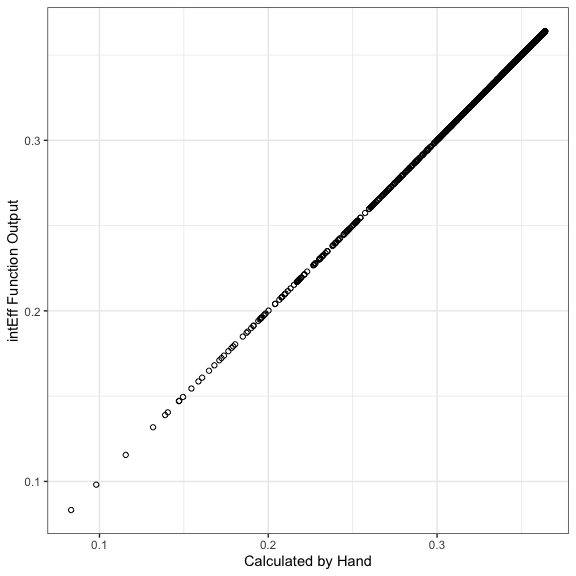So, the byobs list has two elements - the int element holds the interaction effects for each individual observation. The X element holds the original data. These data were used to calculate the interaction effect, except that the variables involved in the interaction effect were changed as we did above. Here, you could plot a histogram of the effects:

i1$byobs$int %>%
ggplot(mapping=aes(x=int_eff)) +
geom_histogram() +
theme_bw() +
labs(x="Interaction Effect")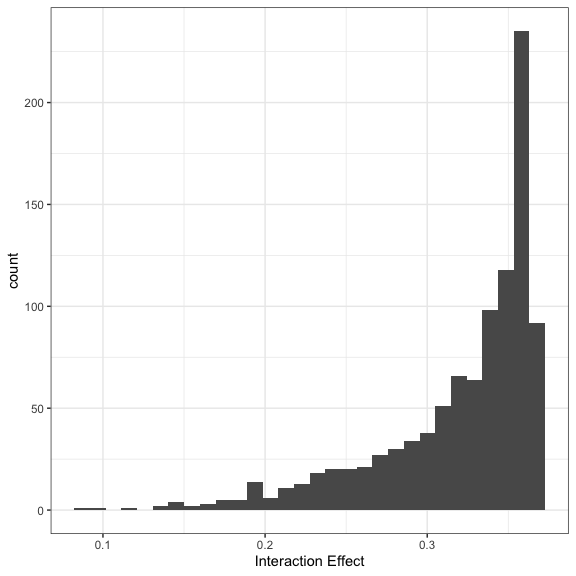In this case, all of the effects are significant, but you could also break these out by significant and not significant effects:

i1$byobs$int %>%
mutate(sig = ifelse(abs(i1$byobs$int$zstat) > 1.96, 1, 0), sig = factor(sig, levels=c(0,1), labels=c("No", "Yes"))) %>% ggplot(mapping=aes(x=int_eff)) + geom_histogram() + theme_bw() + facet_wrap(~sig) + labs(x="Interaction Effect")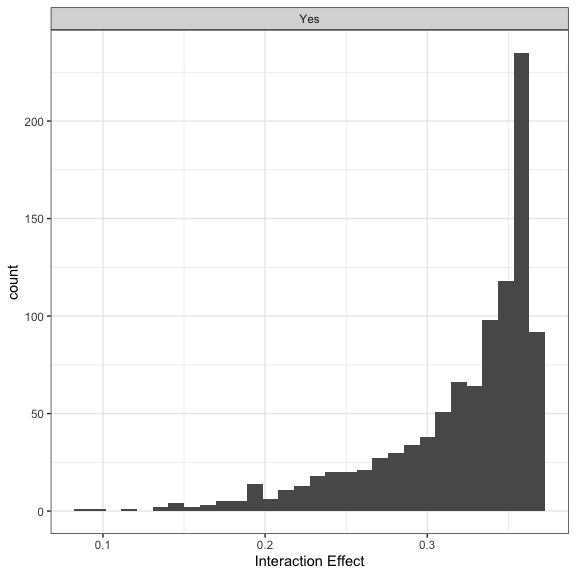I also wrote another function that does this more generally called secondDiff(). This function calculates second differences at user-defined values. The summary function summarises all of the individual second differences like those created above. sd1 <- secondDiff(mod1, c("x1", "x2"), df1) summary(sd1) ## Second Difference Using the Average Marginal Effect Approach ## ## Overall: ## Average Second Difference: -0.318, 95% CI: (-0.424,-0.214) ## ## Individual: ## Significant Negative Individual Second Differences: 1000 ## Significant Positive Individual Second Differences: 0 ## Inignificant Individual Second Differences: 0 These results all tell us about the change in probability when x2 changes from 0 to 1 under two conditions one when x1 is 1 and one when it is 0. For example, the first row of the byobs$int element of the output from intEff():

i1$byobs$int[1,]
##     int_eff    linear      phat se_int_eff    zstat
## 1 0.3584801 0.2141966 0.1384038 0.06166805 5.813061

suggests that for the first observation, as x2 changes from 0 to 1, the first difference is .35 higher when x1 is 1 than when x1 is 0. The atmean element of i1 shows what this difference is at the average of all of the covariates:

i1$atmean ## [] ## int_eff linear phat se_int_eff zstat ## 1 0.3446747 0.4126915 0.6422852 0.05928781 5.813584 ## ##$X
##      (Intercept)   x11   x21          z x11:x21
## [1,]           1 0.518 0.381 0.01450824    0.21

One binary, one continuous.

With one binary and one continuous variable, the Norton, Wang and Ai model would have us calculate the slope of the line tangent to the logit curve for the continuous variable at both of the values of the categorical variable.

First, let’s make the data and run the model:

set.seed(1234)
df2 <- tibble(
x2 = as.factor(rbinom(1000, 1, .5)),
x1 = runif(1000, -2,2),
z = rnorm(1000),
ystar = 0 + as.numeric(x2 == "1") - x1 +
.75*as.numeric(x2=="1")*x1 + z,
p = plogis(ystar),
y = rbinom(1000, 1, p)
)

mod2 <- glm(y ~ x1*x2 + z, data=df2, family=binomial)

Norton, Wang and Ai show that the interaction effect is the difference in the first derivatives of the probability with respect to x1 when x2 changes from 0 to 1. In the following model:

\begin{aligned} log\left(\frac{p_i}{1-p_{i}}\right) &= u_i\\ log\left(\frac{p_i}{1-p_{i}}\right) &= b_0 + b_1x_{1i} + b_2x_{2i} + b_3x_{1i}x_{2i} + \mathbf{Z\theta}, \end{aligned}

The first derivative of the probability with respect to x1 when `x2 is equal to 1 is:

$\frac{\partial F(u_i)}{\partial x_{1i}} = (b_1 + b_3)f(u_i)$

where $$F(u_i)$$ is the predicted probability for observation $$i$$ (i.e., the CDF of the logistic distribution evaluated at $$u_i$$) and $$f(u_{i})$$ is the PDF of the logistic distribution distribution evaluated at $$u_i$$. We could also calculate this for the condition when x2 = 0:

$\frac{\partial F(u_i)}{\partial x_{1i}} = b_1f(u_i)$

In both cases, this assumes that the values of $$\mathbf{x}_{i}$$ are consistent with the condition. For example in the first partial derivative above, $$x_{2i}$$ would have to equal 1 and in the second partial derivative, $$x_{2i}$$ would have to equal zero. We could do this by hand just to see how it works:

X0 <- X1 <- model.matrix(mod2)
## set the conditions for each of the four different
## scenarios above

## x1 = 1, x2=1
X1[,"x21"] <- 1
X1[,"x1:x21"] <- X1[,"x1"]

## x1=1, x2=0
X0[,"x21"] <- 0
X0[,"x1:x21"] <-  0

b <- coef(mod2)

## print the coefficients to show that the two coefficients
## we want are the second and fifth ones.
b
## (Intercept)          x1         x21           z      x1:x21
##   0.3414514  -0.8653259   0.6186529   0.8542471   0.6109292
## calculate the first effect
e1 <- (b + b)*dlogis(X1 %*% b)

## calculate the second effect
e2 <- (b )*dlogis(X0 %*% b)

## calculate the probabilities
eff2 <- e1 - e2

Just like before, we can also estimate the same effect with intEff() and show that the two are the same.

i2 <- intEff(mod2, c("x1", "x2"), df2)
ggplot(mapping=aes(y = i2$byobs$int[,1], x=eff2)) +
geom_point() +
theme_bw() +
labs(x="Calculated by hand", y= "intEff Output")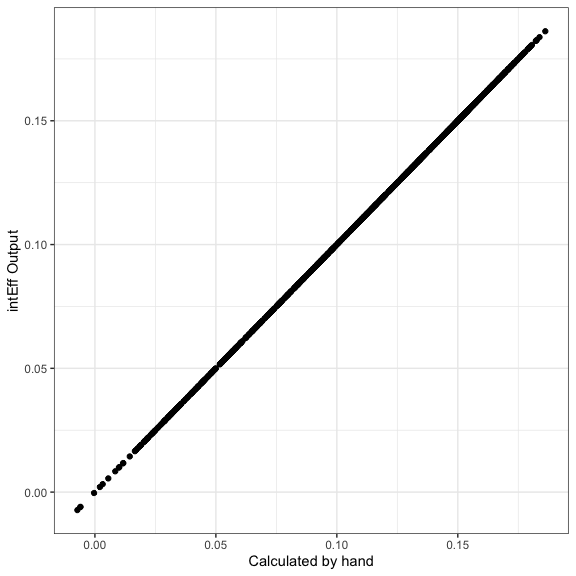Looking at the first line of the output of i2$byobs$int,

i2$byobs$int[1,]
##      int_eff     linear      phat se_int_eff    zstat
## 1 0.04013175 0.07137251 0.1350701  0.0233773 1.716697

We see that the slope of the line tangent to the logit curve when x1 takes on the value of the first observation (1.350536) is 0.04 higher when x2 = 1 than when x2 = 0. We could visualize this as in the figure below. The solid lines are the logit curves and the dotted lines are the lines tangent to the curves at $$x_1 = 1.350536$$. The slope of the blue dotted line is -0.060961 and the slope of the orange dotted line is -0.1010927. You can see that the difference 0.0401317 is the first entry in the int_eff column displayed above.

tmpX1 <- X1[rep(1, 51), ]
tmpX0 <- X0[rep(1, 51), ]
tmpX1[,"x1"] <- tmpX1[,"x1:x21"] <- c(seq(-2, 2, length=50), 1.350536)
tmpX0[,"x1"] <- c(seq(-2, 2, length=50), 1.350536)
tmpX0[,"x1:x21"] <- 0

phat1 <- plogis(tmpX1 %*% b)
phat0 <- plogis(tmpX0 %*% b)
plot.df <- tibble(phat = c(phat0[1:50], phat1[1:50]),
x = rep(seq(-2,2,length=50), 2),
x2 = factor(rep(c(0,1), each=50), levels=c(0,1), labels=c("x2 = 0", "x2 = 1")))

yint1 <- phat1 - e1*tmpX1[51, "x1"]
yint0 <- phat0 - e2*tmpX0[51, "x1"]

plot.df %>%
ggplot(aes(x=x, y=phat, colour = x2)) +
geom_line() +
scale_colour_manual(values=c("#0072B2", "#D55E00")) +
geom_abline(slope=e1, intercept=yint1, colour="#D55E00", lty=3) +
geom_abline(slope=e2, intercept=yint0, colour="#0072B2", lty=3) +
theme_bw() +
labs(x="x1", y="Predicted Probability of y=1", colour="x2")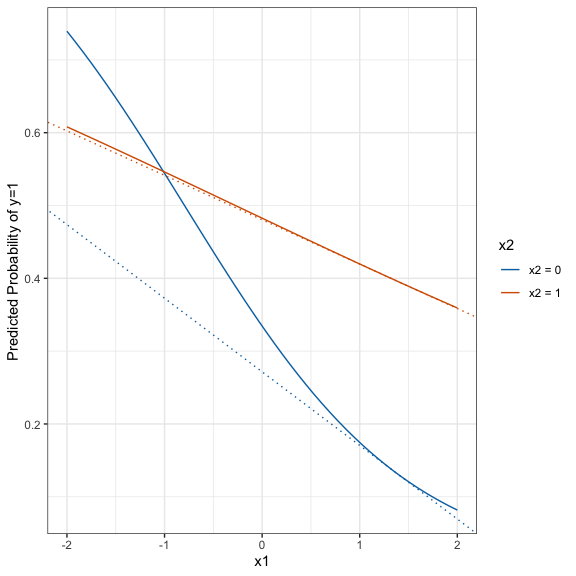From the zstat entry, we see that the effect is not significant. We can plot the effects by significance.

i2$byobs$int %>%
mutate(sig = ifelse(abs(zstat) > 1.96, 1, 0),
sig = factor(sig, levels=c(0,1), labels=c("No", "Yes"))) %>%
ggplot(mapping=aes(x=int_eff)) +
geom_histogram() +
theme_bw() +
facet_wrap(~sig) +
labs(x="Interaction Effect") +
theme(aspect.ratio=1)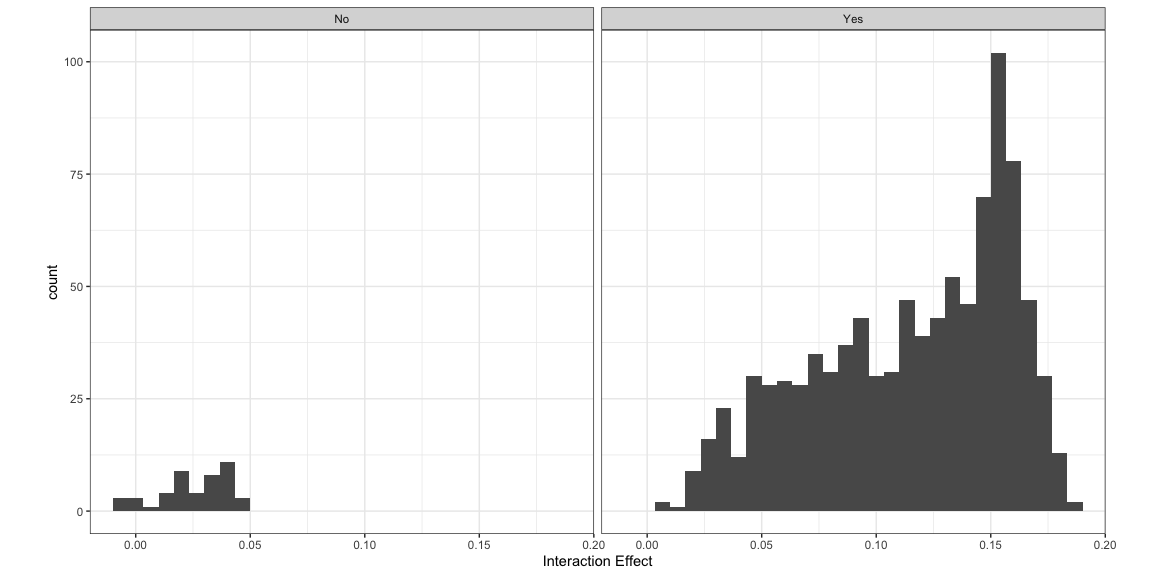Another option here is to do a second difference. Instead of looking at the difference in the slope of the line tangent to the curve for x1, it looks at how the effect of a discrete change in x1 differs across two values of x2. Using the minimum and maximum as the two values to make the change for x1 is the default. But let’s say that we wanted to see how changing x1 from -2 to -1 change the predicted probability of success for x2=0 and x2=1. The result here is the first

\begin{aligned} & \left\{Pr(y=1|x_1=\text{high}, x_2=\text{high}) - Pr(y=1|x_1=\text{low}, x_2=\text{high})\right\} - \\ & \left\{Pr(y=1|x_1=\text{high}, x_2=\text{low}) - Pr(y=1|x_1=\text{low}, x_2=\text{low})\right\} \end{aligned}

s2 <- secondDiff(mod2, c("x1", "x2"), df2, vals=list(x1=c(-2,-1), x2=c("0", "1")))
summary(s2)
## Second Difference Using the Average Marginal Effect Approach
##
## Overall:
## Average Second Difference: 0.079, 95% CI: (0.053,0.110)
##
## Individual:
## Significant Negative Individual Second Differences: 0
## Significant Positive Individual Second Differences: 1000
## Inignificant Individual Second Differences: 0

The summary shows that the change in probabilities is bigger when x2 is low than when x2 is high. This corroborates what we saw in the plot above.

Both Continuous

With two continuous variables using this model:

\begin{aligned} log\left(\frac{p_i}{1-p_{i}}\right) &= u_i\\ log\left(\frac{p_i}{1-p_{i}}\right) &= b_0 + b_1x_{1i} + b_2x_{2i} + b_3x_{1i}x_{2i} + \mathbf{Z\theta}, \end{aligned}

Norton, Wang and Ai show that the cross-derivative, rate of change in the the probabilities as a function of x1 changes as the rate of change in x2 changes.

$\frac{\partial^2 F(u_{i})}{\partial x_{1i} \partial x_{2i}} = b_3f(u_i) + (b1 + b_3x_{2i})(b_2+b_3x_{1i})f'(u_i)$ where $$f'(u_i) = f(u_i)\times (1-2F(u_{i}))$$. We could calculate this “by hand” as:

set.seed(1234)
df3 <- tibble(
x2 = runif(1000, -2,2),
x1 = runif(1000, -2,2),
z = rnorm(1000),
ystar = 0 + as.numeric(x2 == "1") - x1 +
.75*as.numeric(x2=="1")*x1 + z,
p = plogis(ystar),
y = rbinom(1000, 1, p)
)

mod3 <- glm(y ~ x1*x2 + z, data=df3, family=binomial)
X <- model.matrix(mod3)
b <- coef(mod3)
e3 <- b*dlogis(X%*% b) + (b + b*X[,"x2"])*(b + b*X[,"x1"])*dlogis(X%*%b)*(1-(2*plogis(X %*% b)))
i3 <- intEff(mod3, c("x1", "x2"), data=df3)

We can content ourselves that these are the same:

e3
##  0.002296147
i3$byobs$int[1,]
##       int_eff      linear      phat  se_int_eff     zstat
## 1 0.002296147 -0.01145364 0.1248453 0.005366089 0.4278994

So, the effects are the cross-derivative with respect to both x1 and x2. I don’t find this to be a particularly intuitive metric, though we can consider the significance of these values and look at their distribution. I find the second difference metric to be a bit more intuitive because it still gives the difference in change in predicted probabilities for a change in another variable. For example, we could do:

s3 <- secondDiff(mod3, c("x1", "x2"), data=df3, vals =list(x1=c(-1,0), x2=c(-2,2)))
summary(s3)
## Second Difference Using the Average Marginal Effect Approach
##
## Overall:
## Average Second Difference: -0.083, 95% CI: (-0.170,0.003)
##
## Individual:
## Significant Negative Individual Second Differences: 148
## Significant Positive Individual Second Differences: 0
## Inignificant Individual Second Differences: 852

This shows us what happens when we change x1 from -1 to 0 for the two conditions - when x2 is at its minmum versus its maximum. We see that on average the second difference is around -.08 and about 20% of the differences are statistically significant. The average second difference is not, itself, significant. The -0.084 number means the same thing here as it did above. It’s the difference in the first difference of x1 when x2 is high and when x2 is low.